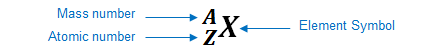# Problem: How many protons and neutrons are in the nucleus of each of the following atoms? In a neutral atom of each element how many electrons are present?d. 133Cs

###### FREE Expert Solution

We’re being asked to determine number o protons, neutrons, and electrons in 133Cs.

An element can be represented as:You can determine the identity of the element using its atomic number:

Atomic number (Z):

represents the identity of the element
reveals the number of protons
in a neutral element:

# of protons = # of electrons

Mass number (A)

reveals the number of protons and neutrons

mass number = # of protons + # of neutrons

Given:

91% (359 ratings)###### Problem Details

How many protons and neutrons are in the nucleus of each of the following atoms? In a neutral atom of each element how many electrons are present?

d. 133Cs

Frequently Asked Questions

What scientific concept do you need to know in order to solve this problem?

Our tutors have indicated that to solve this problem you will need to apply the Subatomic Particles concept. You can view video lessons to learn Subatomic Particles. Or if you need more Subatomic Particles practice, you can also practice Subatomic Particles practice problems.

What professor is this problem relevant for?

Based on our data, we think this problem is relevant for Professor Bass' class at UMBC.

What textbook is this problem found in?

Our data indicates that this problem or a close variation was asked in Chemistry: An Atoms First Approach - Zumdahl Atoms 1st 2nd Edition. You can also practice Chemistry: An Atoms First Approach - Zumdahl Atoms 1st 2nd Edition practice problems.